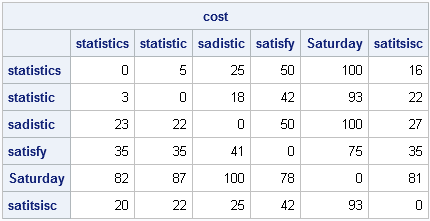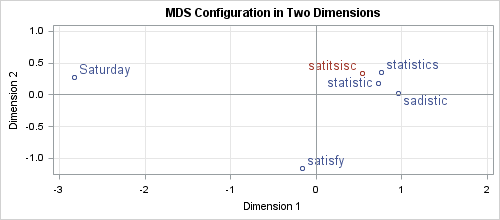When you misspell a word on your mobile device or in a word-processing program, the software might "autocorrect" your mistake. This can lead to some funny mistakes, such as the following:

I hate Twitter's autocorrect, although changing "extreme couponing" to "extreme coupling" did make THAT tweet more interesting. [@AnnMariaStat]

When you type "hte," how does the software know that you probably meant "the"? Even more impressively, when you type "satitsisc" into Microsoft Word, how does it create a list of the words you might have meant, a list that includes "statistics"?

For that matter, have you every mistyped the name of a SAS procedure or an option, only to discover that your program still ran? For example, if you type dat a; run; the program will run, although the SAS log will warn you of the misspelling: "WARNING 14-169: Assuming the symbol DATA was misspelled as dat." How can SAS determine that what you typed is close enough to an actual keyword that it assumes that the keyword was misspelled?

There are various techniques for correcting misspellings, but most of them involve a notion of the distance between words. Given a string of letters, you can define a number that relates how "close" the string is to other words (for example, in a dictionary). The string that is typed is called the keyword. The words in the dictionary are called query words. If the keyword is not in the dictionary, then you can list the query words that are close to the keyword. Often the keyword is a misspelling of one of the words on this list.

Base SAS has several functions that you can use to compare how close words are to each other. In this post, I describe the SPEDIS function, which I assume is an acronym for "spelling distance." Similar SAS functions include the COMPLEV function, the COMPGED function, and the SOUNDEX function.

### What words are close to "satitsisc"

The SPEDIS function computes the "cost" of converting the keyword to a query word. Each operation—deletion, insertion, transposition, and so forth—is assigned a certain cost.

As an example, type the letters "satitsisc" into Microsoft Word and look at the top words that Word suggests for the misspelling. The words are statistics, statistic, and sadistic. The words satisfy and Saturday are not on the list of suggested words. By using the SAS DATA step or the SAS/IML language, you can examine how close each of these words are to the garbled keyword, as measured by the SPEDIS function:

```proc iml; keyword = "satitsisc"; query = {"statistics" "statistic" "sadistic" "satisfy" "Saturday"}; cost = spedis(query, keyword); print cost;```According to the SPEDIS function, the words suggested by MS Word (the first three query words) have a lower cost than satisfy and Saturday, which were not suggested.

The cost returned by the SPEDIS function is not symmetric. For example, the cost of converting sadistic to statistic is different from the cost of converting statistic to sadistic, because inverse operations have different costs. For example, the cost of inserting a letter is different from the cost of deleting a letter.

This lack of symmetry means that the SPEDIS function does not return a true distance between words, although some people refer to the cost matrix as an "asymmetric distance." You can use the SAS/IML language to compute the cost matrix, in which the ijth element is the cost of converting the ith word into the jth word:

```words = query || keyword; n = ncol(words); /* compute (n x n) cost matrix (not symmetric) */ cost = j(n,n,0); do i = 1 to ncol(words); key = words[i]; cost[i,] = spedis(words, key); end; print cost[c=words r=words];```It is possible to visualize the distance between words by using the MDS procedure to compute how to position the words in the plane so that the planar distances most nearly match the (scaled) values in the cost matrix:

```/* write SAS/IML cost matrix to data set */ create cost(type=DISTANCE) from cost[c=words r=words]; append from cost[r=words]; close; quit;   /* find 2D configuration of points */ ods graphics on; proc mds data=cost dimension=2 shape=square; subject words; run;```PROC MDS creates a graph that is similar to the one shown. Notice that the cluster in the upper right consists of the keyword and the words that MS Word suggests. These words are close to the keyword. On the other hand, the two words that were not suggested by MS Word are situated far from the keyword.

This post was inspired by Charlie Huang's interesting article titled Top 10 most powerful functions for PROC SQL. The SPEDIS and SOUNDEX functions are listed in item #6.

If you want to read funny examples of mistakes in text messages that were caused by the autocorrect feature of the iPhone, do an internet search for "funny iPhone autocorrect mistakes." Be aware, however, that many of the examples involve adult humor.

You can use the SPEDIS function to do fuzzy matching, as shown in the following papers:

ShareDistinguished Researcher in Computational Statistics

Rick Wicklin, PhD, is a distinguished researcher in computational statistics at SAS and is a principal developer of SAS/IML software. His areas of expertise include computational statistics, simulation, statistical graphics, and modern methods in statistical data analysis. Rick is author of the books Statistical Programming with SAS/IML Software and Simulating Data with SAS.

1.•